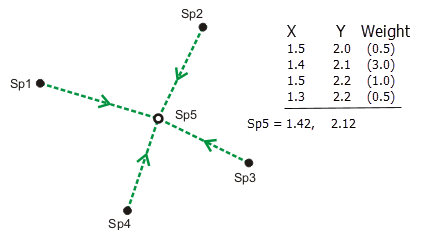A least-squares adjustment uses statistical analysis to estimate the most likely coordinates for connected points in a measurement in a network.

The coordinates of a new point can be uniquely computed by a bearing and a distance from an existing point. However, relying only on the results of a single set of coordinates is risky, since there is no way to tell whether the measurements defining those coordinates are correct. Coordinates computed from measurements from other existing points can be compared with the coordinates computed by the first set of measurements. Generally, the more measurements defining a single point, the more reliable its coordinates are and the more confidence there is in detecting mistake measurements. These additional measurements are called redundant measurements.

All measurements contain some degree of error. Redundant measurements will compute slightly different coordinates for the same point. Since there can only be one coordinate location for a point, best-estimate coordinates for the point can be derived by computing a weighted average of the redundant measurements, with each weight defined by the measurement accuracy. The higher the accuracy of the measurement, the higher its weight and the more influence it will have in computing the best-estimate coordinates of the point.Although the weighted average approach works for computing a single point, it is not sufficient to compute the coordinates for multiple points in a network such as the parcel fabric. The techniques and algorithms in a least-squares adjustment provide the most rigorous and widely accepted solution for computing coordinates in a network of weighted measurements.

To summarize, a least-squares adjustment works as follows:

• Estimates the statistical best-fit solution for coordinates of points in a weighted measurement network.
• Computes a solution by finding a minimum for the sum of the squares of the measurement residuals. A measurement residual is the amount needed to correct a measurement for it to fit into the best-fit solution.
• Is a mathematical procedure based on the theory of probability; estimated coordinates are computed with varying levels of uncertainty.
• Includes statistical tests to analyze and verify adjustment results.

Constrained and free network least squares adjustments

There are many types of least-squares adjustment. The parcel fabric can be adjusted by both constrained and free network least squares adjustments.